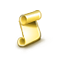Interesting script?
So post a link to it -
let others appraise it

You liked the script? Try it in the MetaTrader 5 terminal# Lot sizing risk based and also converting the currency of the account - script for MetaTrader 4

Views:
7052
Rating:
Published:
2020.04.22 20:14
Updated:
2020.05.03 12:19

I know you're not going to read everything so I'm saying this at the start : IT'S A SCRIPT !  SO IT GOES TO THE SCRIPT FOLDER

So this is it, the following code is based on the following article https://www.fxtrademaker.com/fx_calculation.htm. I had a lot of jobs where the customer was asking me to add a risk based lot sizing (so that when you loose a trade on a SL, you loose only this particular percentage of your account, and not more based on the specific lot sizing), but I never knew the correct formula. Then I discovered that you had 3 cases. (USD is going to be the main currency used)

• First case is the "Direct Rate" if you look at the link above you will see it clearly explained. when you have a direct rate that means that for example your account is in USD and you have a currency like XXXUSD so the formula to calculate the lot size is the following :
```//DIRECT RATES
if(symbol_currency_right==acc_currency){// so when the right currency is the same as your account currency (XXXUSD) in our example
lot_size=money_risk/(dix*sl);//then you divide the money that you want to risk by the Point digits times the size of the lot (standard,micro) times the pips of the SL
}```

• Second case is the "Indirect Rate", it has a type USDXXX just look up the article to know how it is constructed, or analyse the code :
```else if(acc_currency==symbol_currency_left){//GBPXXX
}```
• Last and most complicated case is when the main currency (USD) is not in the symbol at all, you have to pass through a middle currency to convert it correctly so the type would be XXXXXX. The code is a bit more complicated, and an error that I had at the beginning was that the middle symbol wasn't found in the market watch when you tried to get the current price, so first you have to add it
```else if(acc_currency!=symbol_currency_left&&acc_currency!=symbol_currency_right){///XXX XXX
string symbol_2=StringConcatenate(symbol_currency_left,acc_currency);
SymbolSelect(symbol_2,true);

if(r__2==0){
symbol_2=StringConcatenate(acc_currency,symbol_currency_left);
SymbolSelect(symbol_2,true);

}

}```

This code will not work in the strategy tester unless you add a fixed quote of a middle symbol as entry.MA HISTOGRAM

Trend indicatorManual Trading Lightweight Utility

Free utility for manual orders placement.MA HISTOGRAM SEPARATE WINDOW

Trend indicator (separate window)Smoothed Bar to Bar Correlation

The Correlation Indicator provides a smoothed bar to bar correlation value.